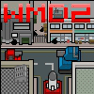Custom Search

# Find Our Cool Math Games to Play by Category:

<<<<< PREVIOUS 7.NS.A.1b GAMES

### 7.NS.A.1b

Understand p + q as the number located a distance |q| from p, in the positive or negative direction depending on whether q is positive or negative. Show that a number and its opposite have a sum of 0 (are additive inverses). Interpret sums of rational numbers by describing real-world contexts.CCSS: 7.NS.A.1b, 7.NS.A.1c
The numbers are rioting.  Help Zero get them under control in this mathematical platformer.  Stuck?  Click on "Walkthrough" in the game to see our video walkthrough.CCSS: 7.NS.A.1b
Ready, aim, fire! Then solve the integer addition, subtraction, multiplication, or division equation to take out the enemy tanks in this version of Weapons of Maths Destruction 2.

<<<<< PREVIOUS 7.NS.A.1c GAMES